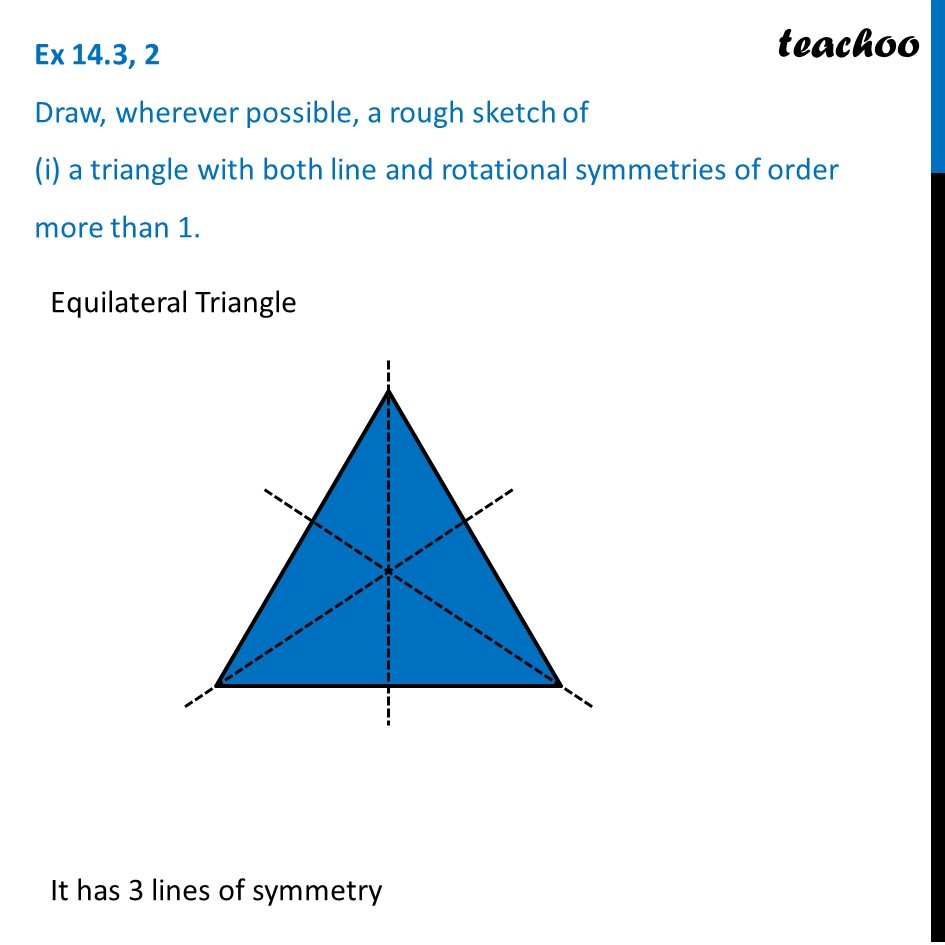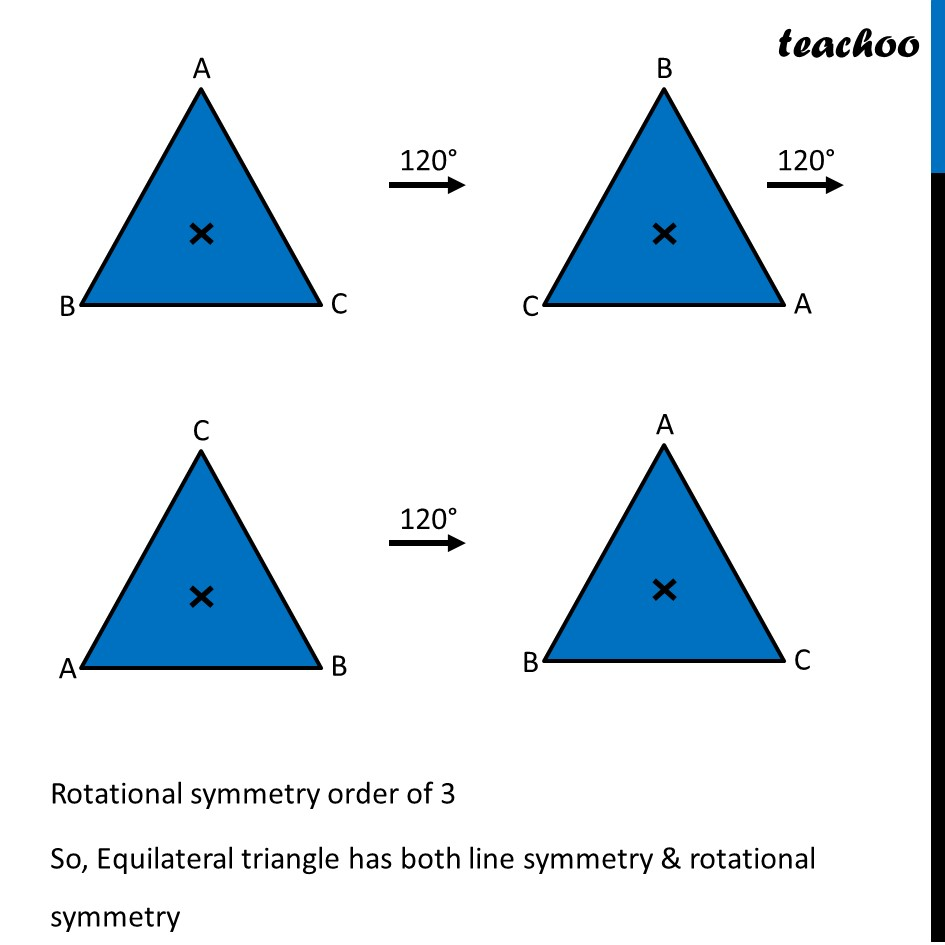Ex 14.3

Chapter 14 Class 7 Symmetry
Serial order wise### Transcript

Ex 14.3, 2 Draw, wherever possible, a rough sketch of (i) a triangle with both line and rotational symmetries of order more than 1.Equilateral Triangle It has 3 lines of symmetry Rotational symmetry order of 3 So, Equilateral triangle has both line symmetry & rotational symmetry